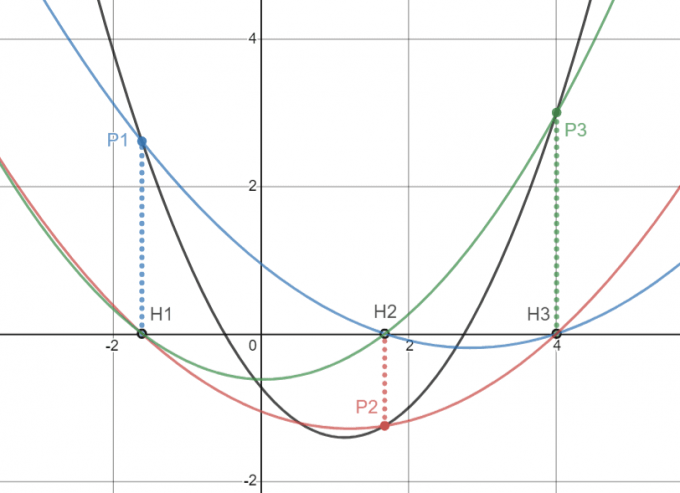# 抱歉，您的浏览器无法访问本站

## 引言

### 符号约定

1. $\prod$ 表示累乘运算，一般其下标会作为累乘的条件；

2. $\sum\limits^n_{i=1}i$ 表示 $1+2+3+\cdots+n$；

3. $f(k)$ 表示关于 $x$ 的函数在 $x=k$ 时的取值；

4. $y=f(x)$ 表示 $y$ 是 $x$ 的函数。

## 问题来源

### 引理

$n+1$ 个点确定一个 $n$ 次的多项式函数。

## 问题求解## 拉格朗日插值法与拉格朗日公式的应用

### 例题

（奥赛经典·奥林匹克数学中的代数问题）$^2$ 设 $a,b,c$ 为非等腰 $\triangle ABC$ 的三边，$S$ 为其面积，求证：$\dfrac{a^3}{(a-b)(a-c)}+\dfrac{b^3}{(b-c)(b-a)}+\dfrac{c^3}{(c-a)(c-b)}>2\times \sqrt{\sqrt{3}^3}\sqrt{S}$。

## 参考文献

 OI Wiki.拉格朗日插值[EB/OL].

 沈文选,张垚,冷岗松．奥赛经典·奥林匹克数学中的代数问题[M]．长沙：湖南师范大学出版社，2009.5 : 322.